## If the mean number of years between asbestos exposure and death is found to be 25 years with a standard deviation of 7 years in a particular

Question

If the mean number of years between asbestos exposure and death is found to be 25 years with a standard deviation of 7 years in a particular county, what is the probability that the mean number of years between asbestos exposure and death will be between 22 and 29 years in a sample of 50 people taken from that community

in progress 0
5 months 2021-08-25T00:11:14+00:00 1 Answers 71 views 0

0.9988 = 99.88% probability that the mean number of years between asbestos exposure and death will be between 22 and 29 years.

Step-by-step explanation:

To solve this question, we need to understand the normal probability distribution and the central limit theorem.

Normal Probability Distribution:

Problems of normal distributions can be solved using the z-score formula.

In a set with mean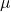and standard deviation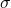, the z-score of a measure X is given by: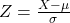The Z-score measures how many standard deviations the measure is from the mean. After finding the Z-score, we look at the z-score table and find the p-value associated with this z-score. This p-value is the probability that the value of the measure is smaller than X, that is, the percentile of X. Subtracting 1 by the p-value, we get the probability that the value of the measure is greater than X.

Central Limit Theorem

The Central Limit Theorem establishes that, for a normally distributed random variable X, with meanand standard deviation, the sampling distribution of the sample means with size n can be approximated to a normal distribution with meanand standard deviation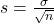.

For a skewed variable, the Central Limit Theorem can also be applied, as long as n is at least 30.

The mean number of years between asbestos exposure and death is found to be 25 years with a standard deviation of 7 years in a particular county

This means that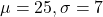Sample of 50:

This means that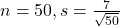What is the probability that the mean number of years between asbestos exposure and death will be between 22 and 29 years?

This is the p-value of Z when X = 29 subtracted by the p-value of Z when X = 22.

X = 29By the Central Limit Theorem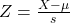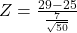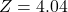has a p-value of 1.

X = 22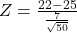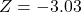has a p-value of 0.0012.

1 – 0.0012 = 0.9988

0.9988 = 99.88% probability that the mean number of years between asbestos exposure and death will be between 22 and 29 years.